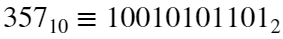# Problem 49835. Decimal to Binary conversion for Large Integers

Decimal integer, a base-10 number we normally use without fractional component, can be represented as binary, a base-2 number composed either 0 or 1. The procedure to convert a decimal integer X to its binary equivalent is as follows:
1. Divide X by 2. The remainder (either 0 or 1) is the first binary value.
2. Divide the quotient of previous step by 2. The remainder is the next binary value.
3. Repeat the process until the quotient cannot be divided anymore and so last binary is found.
As example,through process below:Given a decimal string input x, build a function dectobin(x) that returns its binary equivalent in character array. Unlike built-in dec2bin function, your function should also work for large integers up to thousands number of digits.

### Solution Stats

30.0% Correct | 70.0% Incorrect
Last Solution submitted on Dec 24, 2021

### Community Treasure Hunt

Find the treasures in MATLAB Central and discover how the community can help you!

Start Hunting!